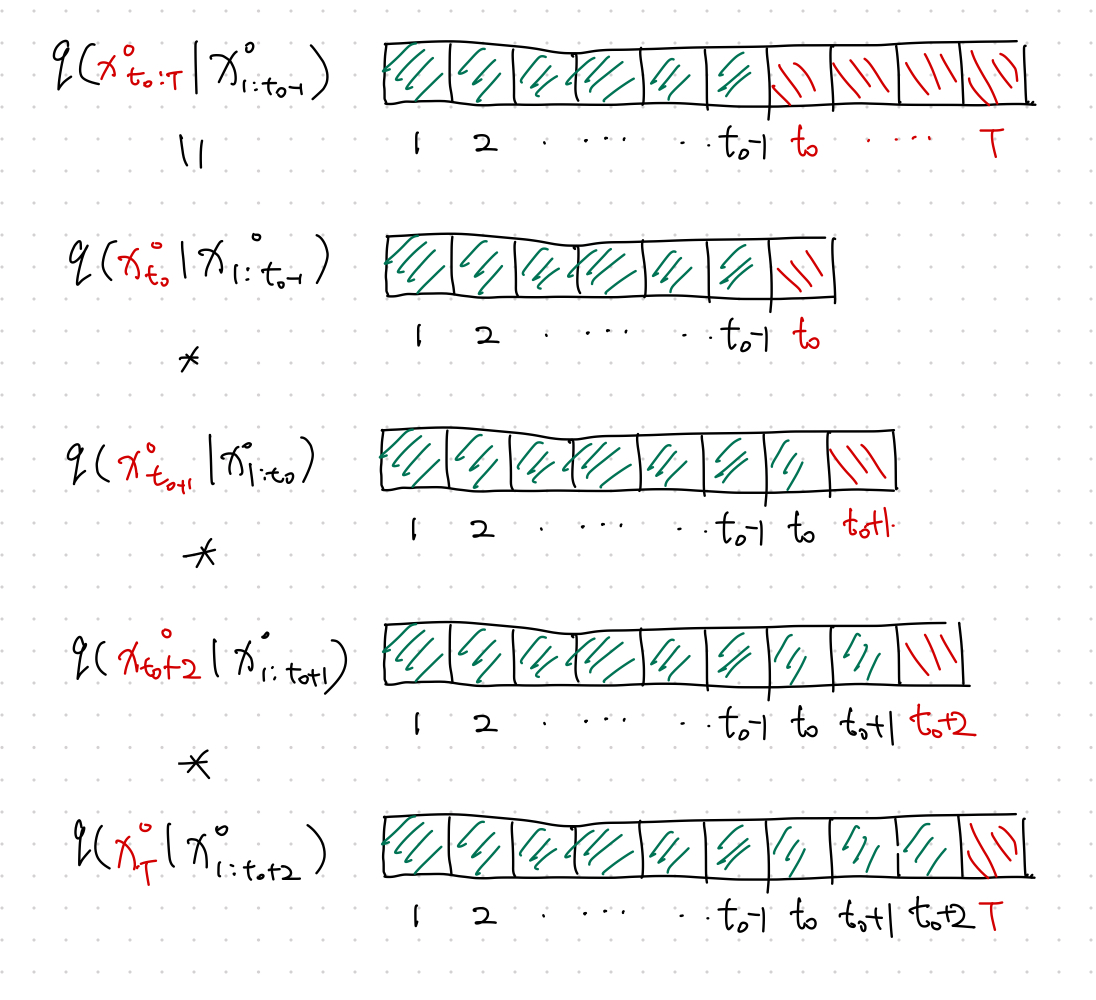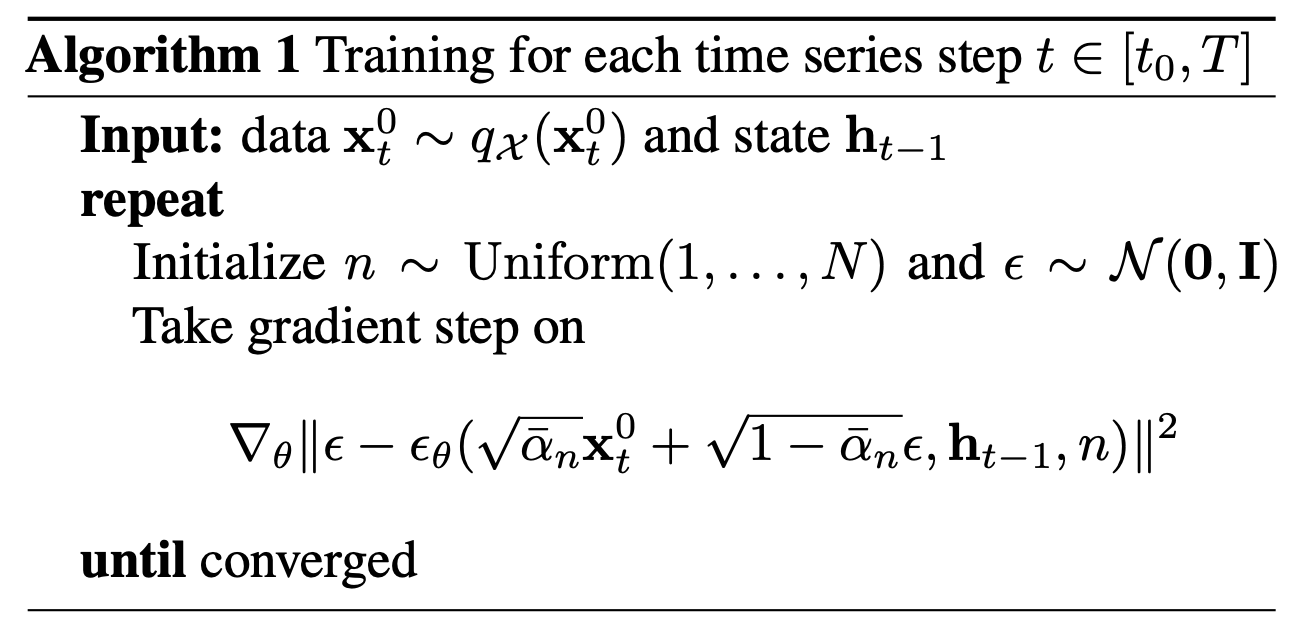Rasul et al., (2021) proposed a probabilistic forecasting model using denoising diffusion models.

## Autoregressive¶

Multivariate Forecasting Problem

Given an input sequence $$\mathbf x_{t-K: t}$$, we forecast $$\mathbf x_{t+1:t+H}$$.

See this section for more Time Series Forecasting Tasks.

Notation

We use $$x^0$$ to denote the actual time series. The super script $${}^{0}$$ will be used to represent the non-diffused values.

To apply the denoising diffusion model in a multivariate forecasting problem, we define our forecasting task as the following autoregressive problem,

$q(\mathbf x^0_{t - K:t} \vert \mathbf x^0_{1:t_0 - 1}) = \Pi_{t=t_0}^T q(\mathbf x^0_t \vert \mathbf x^0_{1:t-1}).$At each time step $$t$$, we build a denoising diffusion model.

## Time Dynamics¶

Note that in the denoising diffusion model, we minimize

$\operatorname{min}_\theta \mathbb E_{q(\mathbf x^0)} \left[ -\log p_\theta (\mathbf x^0) \right]$

The above loss becomes that of the denoising model for a single time step. Explicitly,

$\operatorname{min}_\theta \mathbb E_{q(\mathbf x^0_t )} \left[ -\log p_\theta (\mathbf x^0_t) \right].$

Time dynamics can be easily captured by some RNN. To include the time dynamics, we use the RNN state built using the time series data of the previous time step $$\mathbf h_{t-1}$$1

$\operatorname{min}_\theta \mathbb E_{q(\mathbf x^0_t )} \left[ -\log p_\theta (\mathbf x^0_t \vert \mathbf h_{t-1}) \right].$

Apart from the usual time dimension $$t$$, the autoregressive denoising diffusion model has another dimension to optimize: the diffusion step $$n$$ for each time $$t$$.

The loss for each time step $$t$$ is1

$\begin{equation} \mathcal L_t = \mathbb E_{\mathbf x^0_t, \epsilon, n} \left[ \lVert \epsilon - \epsilon_\theta ( \sqrt{\bar \alpha_n} \mathbf x^0_t + \sqrt{1-\bar \alpha_n}\epsilon, \mathbf h_{t-1}, n ) \rVert^2 \right]. \label{eq:ddpm-loss} \end{equation}$

That being said, we just need to minimize $$\mathcal L_t$$ for each time step $$t$$.

## Training Algorithm¶

The input data is sliced into fixed length time series $$\mathbf x_t^0$$. Since Eq \eqref{eq:ddpm-loss} shows that a loss can be calculated for arbitrary $$n$$ without depending on any previous diffusion steps $$n-1$$, the training can be done by both random sampling in $$\mathbf x_t^0$$ and $$n$$. See Rasul et al. (2021)1.## How to Forecast¶

After training, we obtain the time dynamics encoding $$\mathbf h_T$$, with which the denoising steps can be calculated using the reverse process

$\mathbf x^{n-1}_{T+1} = \frac{1}{\alpha_n} \left( \mathbf x^n_{T+1} - \frac{\beta_n}{1 - \bar\alpha_n} \epsilon_\theta( \mathbf x^n_{T+1}, \mathbf h_{T}, n ) \right) + \sqrt{\Sigma_\theta} \mathbf z,$

where $$\mathbf z \sim \mathcal N(\mathbf 0, \mathbf I)$$.

For example,

$\mathbf x^{0}_{T+1} = \frac{1}{\alpha_1} \left( \mathbf x^1_{T+1} - \frac{\beta_1}{1 - \bar\alpha_1} \epsilon_\theta( \mathbf x^1_{T+1}, \mathbf h_{T}, 1 ) \right) + \sqrt{\Sigma_\theta} \mathbf z.$

## It is Probabilistic¶

The quantiles is calculated by repeating many times for each forecasted time step1.

## Code¶

An implementation of the model can be found in the package pytorch-ts 2.

1. Rasul K, Seward C, Schuster I, Vollgraf R. Autoregressive Denoising Diffusion Models for Multivariate Probabilistic Time Series Forecasting. arXiv [cs.LG]. 2021. Available: http://arxiv.org/abs/2101.12072

2. Rasul K. PyTorchTS. https://github.com/zalandoresearch/pytorch-ts

Contributors: LM